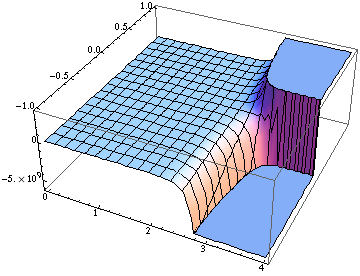## Friday, October 09, 2009

Upon popular request, I wrote a small script to download all tweets of a given twitter id. Have fun!

## Sunday, March 01, 2009

### Posts disappearing

As I have noted earlier, some posts by John Baez and others on certain crackpots having their own Elsevier journal to dump their E-infinity weirdness have disappeared from the net. Luckily, one brave soul keeps copies of this stuff.

Now, I had to learn, that they completely overtook the discussion section of the online version on a Scientific American article on dynamical triangulations and what is even more disturbing, made the weekly quality newspaper "Die Zeit" take down the online version of an article reporting these issues (in German). I would have thought it would take a lot to make a respectable paper with a law department to commit this kind of self censorship.

## Tuesday, February 17, 2009

### Initial data for higher order equations of motion

Over lunch, today, we had a discussion on higher order quantum corrections in the effective action. You start out with a classical action that only contains terms with up to two derivatives. This corresponds to equations of motion that are second order in time. As such, for the physical degrees of freedom (but I want to ignore a possible gauege freedom here) you then have to specify the field and its time derivate on a Cauchy surface to uniquely determine the solution.

Loop corrections, however, tyically lead to terms with any number of derivatives in the effective action. Corresponding equations of motion allow then for more initial data to be specified. The question then is what to do with the unwanted solutions. If you want this is the classical version of unitarity.

Rather than discussing higher derivative gravity (where our lunch discussion took off) I would like to discuss a much simpler system. Say, we have a one dimensional mechanical system and the classical equation of motion is as simple as it can get, just. To simplify things, this is only first order in time and I would like to view a second order term already as "small" correction. The higher order equation would then bewith small.

To find solutions, one uses the ansatzand finds. For small, the two exponents behave aswhich blows up andwhich approaches the solution of the "classical equation".

The general solution is a linear combination of the two exponential functions. We see that the solution blows up over a time-scale ofunless the initial data satisfies the classical equation.

We can turn this around and say that if the classical equation is satisfied initially, we are close to the classical solution for long time (it's not exactly the same sincediffers from the "classical exponent"by orderterms. For other initial data, the solution blows up exponentially on a "quantum time" inversely proportional to the small parameter.This plot showsfor. On the axis that goes into the picture there is a parameter for the initial conditions which isfor data satisfying the classical equation initially. You can see that this parameter determines ifgoes toorover short time. Only the classical initial data stays small for much longer.

Unfortunately, this still leaves us with the question of why nature chooses to pick the "classical" initial data and seems not to use the other solutions. In the case of higher order gravity there is of course an anthropic argument that suggests itself but I would rather like to live without this. Any suggestions?